U.S. Department of Transportation
1200 New Jersey Avenue, SE
Washington, DC 20590
202-366-4000

Federal Highway Administration Research and Technology
Coordinating, Developing, and Delivering Highway Transportation Innovations

 REPORT This report is an archived publication and may contain dated technical, contact, and link information
 Publication Number:  FHWA-HRT-12-030    Date:  August 2012
 Publication Number: FHWA-HRT-12-030 Date: August 2012

# Estimation of Key PCC, Base, Subbase, and Pavement Engineering Properties From Routine Tests and Physical Characteristics

PDF files can be viewed with the Acrobat® Reader®

# CHAPTER 5. MODEL DEVELOPMENT (12)

## PCC Elastic Modulus Models

As with flexural strength, the development of PCC elastic modulus models required a detailed data assembly appropriate for statistical analyses. These analyses also entailed the validation of existing models as well as the development of new models. Model forms of existing models were utilized to fit the data assembled in this study for the validation. The development of new models was not as straightforward as for the other PCC models, primarily because the physical characteristics of a PCC mixture that affect the elastic modulus are not fully captured within the data used for building a mathematical relationship.

The following are key points to note about flexural strength data:

• Elastic modulus test results were available for SPS and GPS sections.
• Test data were collected at 28 days and 1 year for most of the SPS sections. In addition, two SPS-2 sections and two SPS-8 sections had data at 10 and 4.5 years, respectively. Compressive strength data also were present for corresponding specific ages. The data were matched for these specific age categories.
• SPS-2 sections representing the two mix designs targeting low (550 psi) and high (900 psi) strengths showed marginal differences in the elastic modulus results.
• After data reduction, a majority of the observations used in the statistical models belonged to GPS sections. Multiple sections at SPS-2 sites were averaged to produce a single observation, thereby reducing the number of SPS-2 observations.

### Validation of Existing Models

Existing models correlate elastic modulus to compressive strength and unit weight. Although more recent models have attempted to introduce lithological type of the coarse aggregate and admixture parameters, within the context of this study, there were not adequate data to validate them. Figure 186 to figure 189 summarize the regressed models using LTPP data.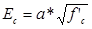#### Figure 186. Equation. Ec as a function of square root of compressive strength.

Regressed coefficients for figure 186 are as follows:

• a = 55,294.

Regression statistics for figure 186 are as follows:

• N = 514.
• R2 = 11.8 percent.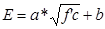#### Figure 187. Equation. Model form for E as a function of compressive strength with slope and intercept.

Regressed coefficients for figure 187 are as follows:

• a = 31,624.6.
• b = 2,013,192.

Regression statistics for figure 187 are as follows:

• N = 514.
• R2 = 11.8 percent.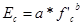#### Figure 188. Equation. Ec.

Regressed coefficients for figure 188 are as follows:

• a = 388,082.
• b = 0.2809.

Regression statistics for figure 188 are as follows:

• N = 514.
• R2 = 12.3 percent.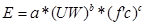#### Figure 189. Equation. E as function of unit weight and compressive strength.

Regressed coefficients for figure 189 are as follows:

• a = 80,849.3.
• b = 0.3648.
• c = 0.2527.

Regression statistics for figure 189 are as follows:

• N = 514.
• R2 = 10.8 percent.

The quality of prediction in the validated models is poor, as indicated by the R2 values in figure 186 through figure 189. The predicted versus measured plots for these models have not been included in this report, but they show higher predictions for the lower modulus values and lower predictions for the higher modulus values. This trend is common with elastic modulus models, especially considering that the data used in this study were not generated from controlled laboratory experiments. Also, while compressive strength is the most commonly used strength parameter and correlations with the compressive strength can be implemented most easily, there is an inherent drawback in correlating modulus to compressive strength. Modulus does not test the material to its limits, and, as discussed in chapter 2, it is more indicative of the elastic deformational characteristics of the material. Additionally, it often captures other ITZ characteristics and can be a good indicator of concrete durability. Finally, the data contain modulus measured at a wide range of ages. The new models developed therefore utilized other mix parameters that impact modulus including age.

### Elastic Modulus Model 1: Model Based on Aggregate Type

Several mix design parameters were evaluated for the elastic modulus model 1 in addition to the compressive strength and unit weight parameters. The model development efforts particularly focused on the aggregate type, given the strong influence of the aggregate hardness on the measured elastic modulus values.

This model utilizes a subset of the data used in the model validation process and has only 71 data points compared to 514 observations in the validation models. This was primarily due to the inclusion of aggregate type in the relationship. Coarse aggregate type information is present in both the materials tables and the CTE tables of the LTPP database. Several data inconsistencies were found in comparing the aggregate types listed in these two tables; therefore, for the development of CTE models (discussed later in this chapter), only those cases with the same aggregate type in both tables were used. In other words, the two tables were used to validate the data against each other. This vastly reduced the dataset used. The dataset included both SPS and GPS sections; however, a majority of the data used in this model belonged to GPS sections.

A nonlinear analysis was performed to establish the following equation: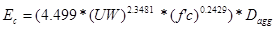#### Figure 190. Equation. Prediction model 9 for Ec.

Where:

Ec = PCC elastic modulus, psi.

UW = Unit weight, lb/ft3.

f'c = Compressive strength.

Dagg = Regressed constant depending on aggregate type as follows:

• = 10 for andesite.
• = 0.9286 for basalt.
• = 1.0079 for chert.
• = 0.9215 for diabase.
• = 1.0254 for dolomite.
• = 0.8333 for granite.
• = 1.0 for limestone.
• = 0.9511 for quartzite.
• = 1.0 for sandstone.

The development of the model required the use of a model form which accommodates aggregate type as categorical variables (assigned values of 1,0). The values for Dagg were initialized to 1.0 at the start of the analyses and allowed to iteratively determine individual values for each aggregate type. The model had 71 observations, an R2 value of 35.8 percent, and an RMSE value of approximately 500,000 psi. The nonlinear analyses results are presented in table 39.

#### Table 39. Regression statistics for elastic modulus model based on aggregate type.

 Parameter Comment Estimate Standard Error Approximate 95 Percent Confidence Limits a No comment 4.499 18.6844 -32.8506 41.8485 b No comment 2.3481 0.8998 0.5495 4.1468 c No comment 0.2429 0.1224 -0.00173 0.4875 d Dagg for andesite 1 N/A N/A N/A e Dagg for basalt 0.9286 0.0956 0.7374 1.1197 f Dagg for chert 1.0079 0.0863 0.8354 1.1803 g Dagg for diabase 0.9215 0.1858 0.5501 1.2928 h Dagg for dolomite 1.0254 0.0624 0.9006 1.1501 i Dagg for granite 0.8333 0.0624 0.7085 0.9581 j Dagg for limestone 1 N/A N/A N/A k Dagg for quartzite 0.9511 0.1082 0.7349 1.1674 l Dagg for sandstone 1 N/A N/A N/A

N/A = Not applicable.

The model statistics for table 39 are as follows:

• RMSE = 499,856 psi.
• R2 = 0.3582 percent.
• N = 71.

The model form described for the statistics presented in this table is as follows: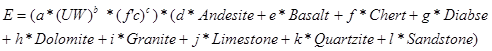#### Figure 191. Equation. E.

Where:

d through l were iteratively determined through the nonlinear process, and andesite, basalt, chert, diabase, dolomite, granite, limestone, quartzite, and sandstone are categorical variables with values 0, 1.

Table 39 indicates that the factor that accounts for the aggregate type, Dagg, has a value of 1.0 for andesite, limestone, and sandstone. Basalt, diabase, granite, and quartzite have lower Dagg values and therefore lower modulus values than mixes using andesite, limestone, and sandstone aggregates. Likewise, chert and dolomite have higher values. Also evident from table 39 is that to a very small extent, the statistical optimization has been compromised (note significance of parameters a and c are not within limits) in the interest of developing a model with variables relevant to elastic modulus predictions. Table 40 provides details of the range of data used to develop the model. The R2 value is reasonable and therefore presented as a feasible model. Figure 192 and figure 193 show the predicted versus measured plot and the residual plot, respectively.

#### Table 40. Range of data used for elastic modulus model based on aggregate type.

 Parameter Minimum Maximum Average Compressive strength 1,990 11,310 7,550 Unit weight 137 156 146 Elastic modulus 1,450,000 6,800,000 4,629,646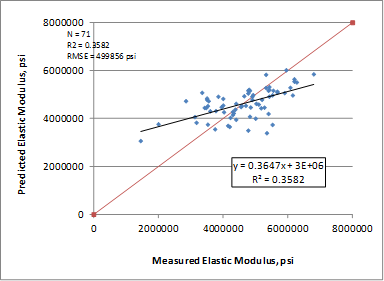#### Figure 192. Graph. Predicted versus measured for elastic modulus model based on aggregate type.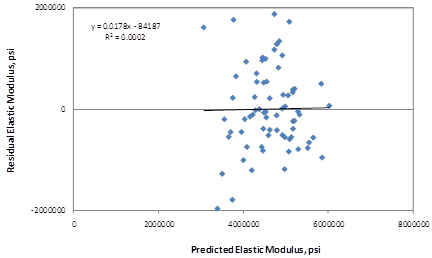#### Figure 193. Graph. Residual errors for elastic modulus model based on aggregate type.

Federal Highway Administration | 1200 New Jersey Avenue, SE | Washington, DC 20590 | 202-366-4000
Turner-Fairbank Highway Research Center | 6300 Georgetown Pike | McLean, VA | 22101